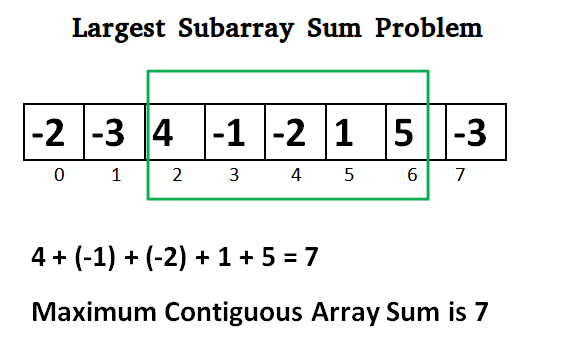Open in App
Not now

# Javascript Program for Largest Sum Contiguous Subarray

• Last Updated : 09 Dec, 2021

Write an efficient program to find the sum of contiguous subarray within a one-dimensional array of numbers that has the largest sum.```Initialize:
max_so_far = INT_MIN
max_ending_here = 0

Loop for each element of the array
(a) max_ending_here = max_ending_here + a[i]
(b) if(max_so_far < max_ending_here)
max_so_far = max_ending_here
(c) if(max_ending_here < 0)
max_ending_here = 0
return max_so_far```

Explanation:
The simple idea of Kadane’s algorithm is to look for all positive contiguous segments of the array (max_ending_here is used for this). And keep track of maximum sum contiguous segment among all positive segments (max_so_far is used for this). Each time we get a positive-sum compare it with max_so_far and update max_so_far if it is greater than max_so_far

```    Lets take the example:
{-2, -3, 4, -1, -2, 1, 5, -3}

max_so_far = max_ending_here = 0

for i=0,  a =  -2
max_ending_here = max_ending_here + (-2)
Set max_ending_here = 0 because max_ending_here < 0

for i=1,  a =  -3
max_ending_here = max_ending_here + (-3)
Set max_ending_here = 0 because max_ending_here < 0

for i=2,  a =  4
max_ending_here = max_ending_here + (4)
max_ending_here = 4
max_so_far is updated to 4 because max_ending_here greater
than max_so_far which was 0 till now

for i=3,  a =  -1
max_ending_here = max_ending_here + (-1)
max_ending_here = 3

for i=4,  a =  -2
max_ending_here = max_ending_here + (-2)
max_ending_here = 1

for i=5,  a =  1
max_ending_here = max_ending_here + (1)
max_ending_here = 2

for i=6,  a =  5
max_ending_here = max_ending_here + (5)
max_ending_here = 7
max_so_far is updated to 7 because max_ending_here is
greater than max_so_far

for i=7,  a =  -3
max_ending_here = max_ending_here + (-3)
max_ending_here = 4```

Program:

## Javascript

 ``

Output:

`Maximum contiguous sum is 7`

Another approach:

## Javascript

 ``

Time Complexity: O(n)
Following is another simple implementation suggested by Mohit Kumar. The implementation handles the case when all numbers in the array are negative.

## Javascript

 ``

Output:

`Maximum contiguous sum is 7`

To print the subarray with the maximum sum, we maintain indices whenever we get the maximum sum.

## Javascript

 ``

Output:

```Maximum contiguous sum is 7
Starting index 2
Ending index 6```

Kadane’s Algorithm can be viewed both as a greedy and DP. As we can see that we are keeping a running sum of integers and when it becomes less than 0, we reset it to 0 (Greedy Part). This is because continuing with a negative sum is way more worse than restarting with a new range. Now it can also be viewed as a DP, at each stage we have 2 choices: Either take the current element and continue with previous sum OR restart a new range. These both choices are being taken care of in the implementation.

Time Complexity: O(n)

Auxiliary Space: O(1)

Now try the below question
Given an array of integers (possibly some elements negative), write a C program to find out the *maximum product* possible by multiplying ‘n’ consecutive integers in the array where n ≤ ARRAY_SIZE. Also, print the starting point of the maximum product subarray.

Please refer complete article on Largest Sum Contiguous Subarray for more details!

My Personal Notes arrow_drop_up
Related Articles